# Wall invariant

An element of the Wall group, representing the obstruction to the surgery of a bordism to a simple homotopy equivalence.

Letbe a finite Poincaré complex,a fibre bundle overand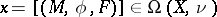a bordism class, whereis the formal dimension ofand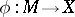has degree 1. This mapping can always be represented by an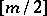-connected mapping using a finite sequence of surgeries. Let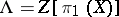be a group ring and letbe the involution on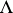given by the formula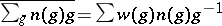, where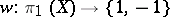is defined by the first Stiefel–Whitney class of. Put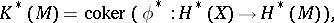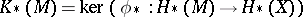(coefficients in). The involution is an anti-isomorphism and there is defined the Wall group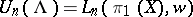.

Suppose now that. Then in the stable free-module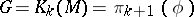one can choose a basis, and Poincaré duality induces a simple isomorphism, where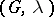is a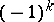-form. One therefore obtains the class.

Suppose next that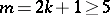. One can choose generators in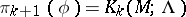so that they represent the imbeddings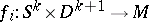, with non-intersecting images, and these images are connected by paths with a base point. Put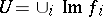,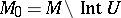. Since, one may replaceby a homotopy and suppose that. Becauseis a Poincaré complex, one can replaceby a complex with a unique-cell, i.e. one has a Poincaré pairand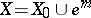. By the choice of a suitable cellular approximation one obtains a mapping for the Poincaré triad of degree 1: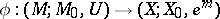. Consequently one has the diagram of exact sequences: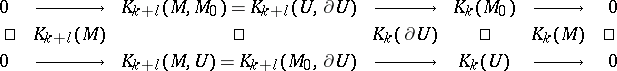Moreover, one has a non-degenerate pairing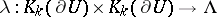, where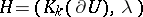is a quadratic-form while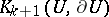and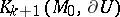define its Lagrange planesand. Then.

The elementsdefined above are called the Wall invariants. An important property is the independence ofon the choices in the construction and the equivalence of the equationto the representability of the class as a simple homotopy equivalence, cf. .